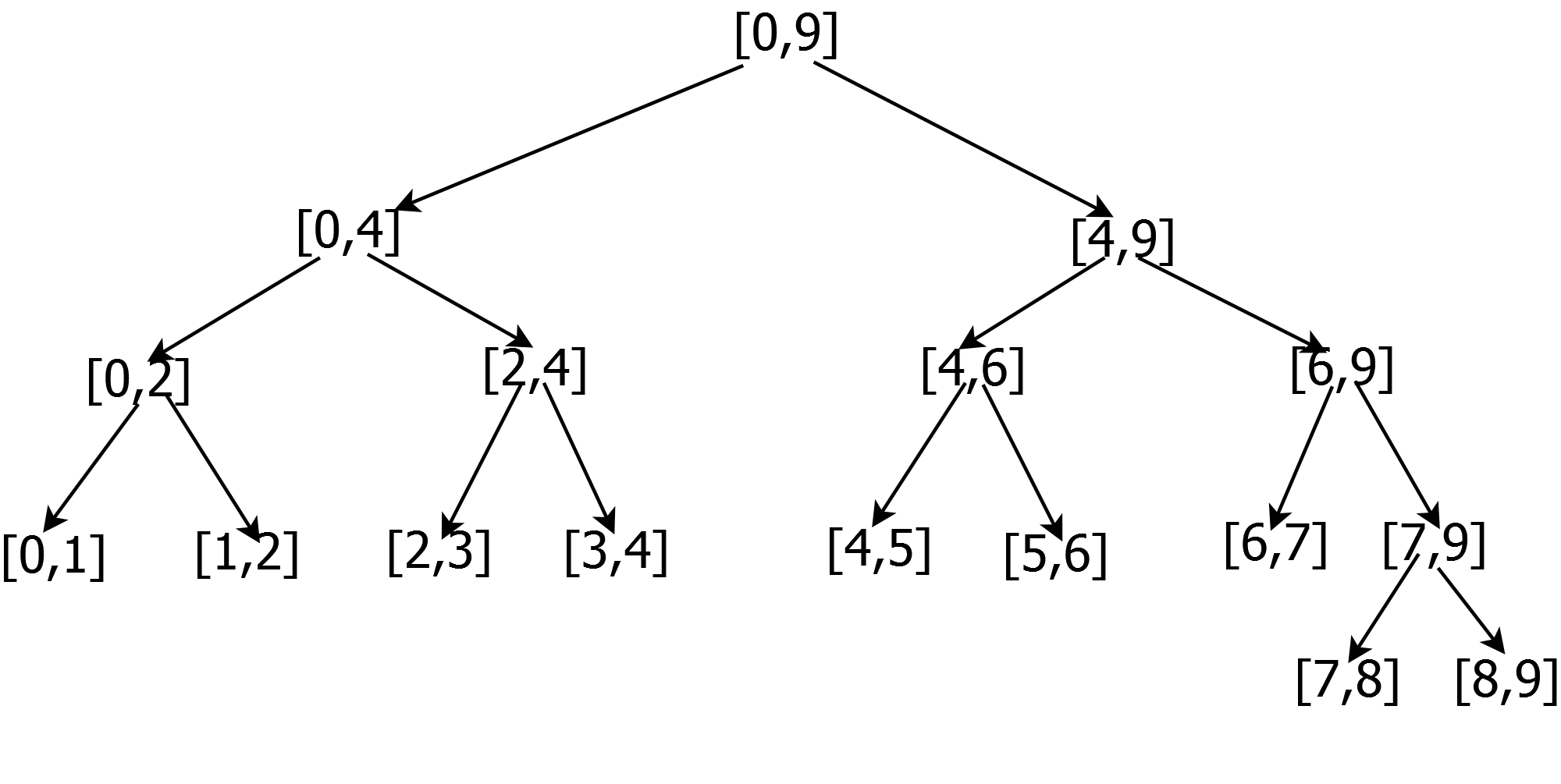Saturday, June 1, 2013

Cumulative Sums of elements in an array[Binary Indexed Tree]

Given a array and with below possible operations
Update: Add a value to a index a
Query: Get a cumulative sum from a range a to b
What is the data structure you would employ and what will be the time complexity of the update and query operations.

Approach:
The basic idea of any of the algorithm is to store/update the sum of the subparts and compute the sum in a range by adding up the relevant subparts.So for e.g a common data structure Segment tree creates a tree where the parent stores the sum of the child elements.

So each node in the tree takes care of the sum in a particular range.Once you create a static Segment tree the update and the query operation should be easy.

Can we avoid the extra space incurred and rather use the given array elements as the nodes to hold the sum in a range.
Infact a famous data structure Binary Index Tree(also known as Fenwick tree) works on the same principle and is relatively simple to code and debug.

So how do one assign the parent child relationship in the tree. Since now there are no internal node to hold the sum of a range like (0,4) we can infact use the 4th index to hold the sum of (0, 4), the same is true for the
3 holding the sum of (3,3)
10 holding the sum of (9,10)
12 holding the sum of (9,12)
16 holding the sum of (0,16)
So to generalize each index index holds the sum of elements in range
((index - 2^r + 1) , index) where r is the right most bit postion set in index.

I would strongly suggest to go over the above logic once more and write a pseudo code for the update query.

Kudos if you were successful to write the working piece of the code.

So how do we add a delta to a index, we need to update all the elements which are using its value.
Also to extract the rightmost bit we can use
n & -(n)    //Proof is left to reader :)
Below is the terse piece of code which updates the delta at a particular index

```void update(int index ,int delta){
while (index <= N){             // N is the total number of elements in the array
A[index] += delta;
index += (index & -index);
}
}
```
To initialize the array we can intialize all the array elements to 0 and iteratively call the update function for each of the index.

To find the sum of elements in the range (0, index), the below function can be used
```int query(int index){
int sum = 0;
while (index > 0){
sum += A[index];
index -= (index & -index);
}
return sum;
}
```

To find the sum in a given range (a,b), we can compute it by
```int sum = query(b) - query(a-1);
```

Finally the time complexity of each of the update, query is O(log2(N))